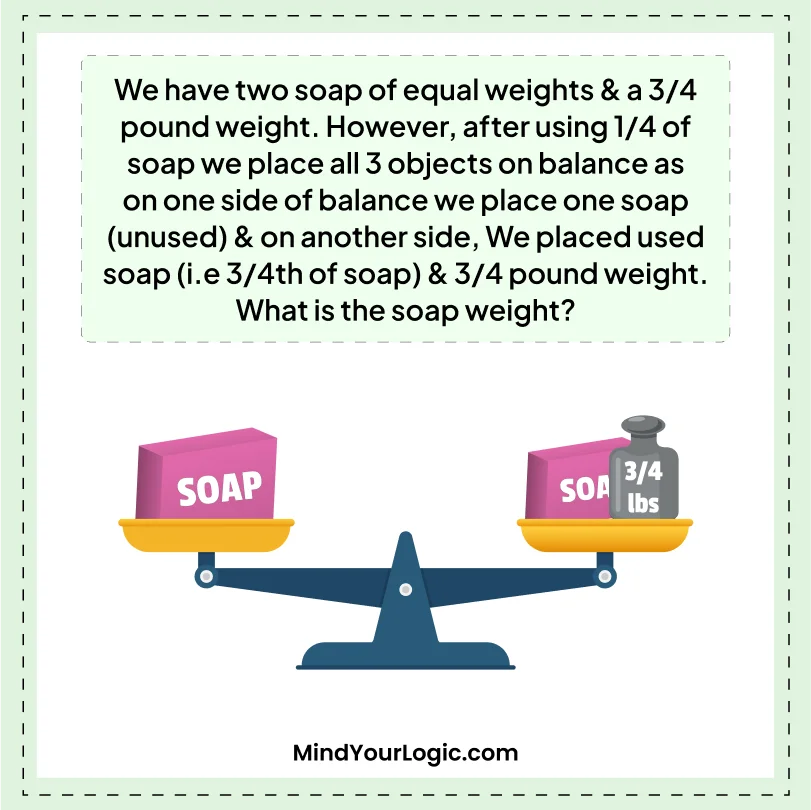# Two soap of equal weights - Math Riddle

###### 114.Math Riddles
`We have two soap of equal weights & a 3/4 pound weight. However, after using 1/4 of soap we place all 3 objects on balance as on one side of balance we place one soap (unused) & on another side, We placed used soap (i.e 3/4th of soap) & 3/4 pound weight. What is the soap weight?`
`What is the weight of soap?`• `3 lbs.`
```Explanation :

If the balance was equal when one soap was on one side and the other soap and a 3/4 pound weight were on the other side, we can use this information to find the weight of the soap.

Let's call the weight of the soap "x". Then, when one soap was on one side, the weight on the other side was (3/4) + (3/4) * x. Since the balance was equal, the weight of the two sides must be equal, so we can set them equal to each other and solve for x:

x = (3/4) + (3/4) * x

Solving for x, we get:

x = 3/4 + 3/4x

Subtracting 3/4x from both sides:

1/4x = 3/4

Dividing both sides by 1/4:

x = 3

So the weight of each soap is 3 pounds.```